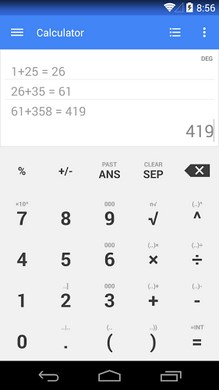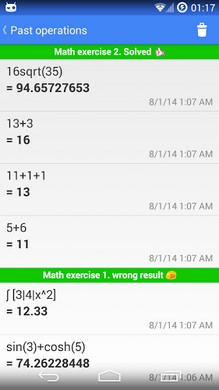# One Calculator APKOne Calculator is a fully featured scientific calculator with a simple and clean interface, perfectly integrated with Google Material Design. Supplying different calculus modalities, One Calculator is suitable for solving easy common calculus and more complex mathematical calculations.

One Calculator characteristics:
. Basic Arithmetic
. Definite integrals
. Trigonometry (sin, cos, tan, arcsin, arccos, arctan)
. Complex numbers
. RPN calculations (Reverse Polish notation/postfix notation)
. Matrix calculus
. Measurement unit conversion
. Graphs for X,Y equations
. Conversion between decimal, hexadecimal, octal and binary
. Intreactive chronology of operations
. Specialized warning of errors
. Possibility to modify the calculator interface
. Support for tablet and smartphones
Help me to translate the app: Ackuna.com/translate-/one-calculator-3/

Size : 2.9M
Current Version : 2.2
Requires Android : 4.0 and up
Offered By : Pierfrancesco SoffrittiThis site uses Akismet to reduce spam. Learn how your comment data is processed.